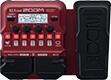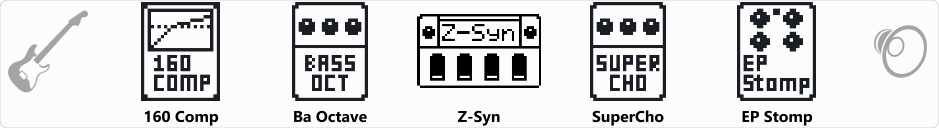# Synth! BOY!

Discussion in 'Zoom B1/B1X Four' started by Kalmek, Dec 16, 2021.

1. Synth! BOY!Device: Zoom B1X Four
Firmware: 2.00

Noodle sample here.

Name on device: Synth!
Optimized for: Phones/Speaker

Effects chain:Phat Synth sound, with option to turn on BassOctave (2nd fx) for even phatter sound - use at own risk! (And also see where limits of Zoom AND your sub speakers are)

Effect: "160 Comp" (Dynamics), active - "yes"
"Threshold" = -9
"Ratio" = 2.1
"Knee" = HARD
"Volume" = 54

Effect: "Ba Octave" (Modulation), active - "yes"
"Oct" = 56
"Lo" = 4
"Hi" = 4
"Dry" = 33

Effect: "Z-Syn" (Sfx), active - "yes"
"FREQ" = 10
"Range" = 3
"Decay" = 29
"RESO" = 0
"Wave" = 0
"Tone" = 5
"BAL" = 81
"VOL" = 29
"Frequency" = 0
"Range" = 0
"Decay" = 0
"Resonance" = 0
"Wave" = SAW
"Tone" = 0
"Balance" = 0
"Volume" = 0

Effect: "SuperCho" (Modulation), active - "yes"
"Depth" = 32
"Rate" = 86
"Tone" = 35
"Mix" = 42

Effect: "EP Stomp" (Overdrive / Distortion), active - "yes"
"Gain" = 63
"Bass" = 7
"Treble" = 0
"Volume" = 15
Patch Volume: 98